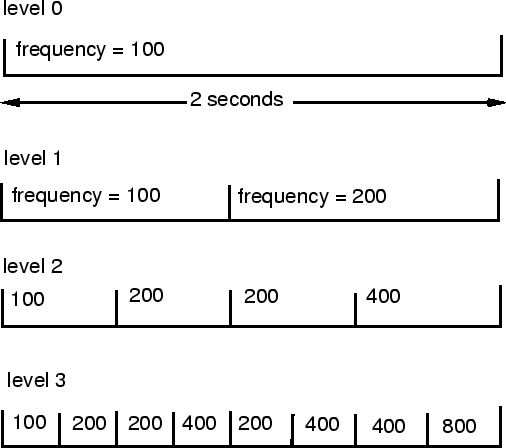Next: The Synthesis Language Up: Synthesis Method Previous: Synthesis Method   Contents

In this method the user defines a hierarchy of structures to create the sound. The hierarchy can contain recursive elements. This structure is defined by a series of factor arrays. ``Time'' is the factor which defines the segmentation of time into different cells. All parameters are developed by applying the current level factors to higher level values. Thus, a segment of sound becomes a multi-layer collection of cells organized in time, while a series of parameters are active for the duration of every cell. It is useful to explain the synthesis method with a simple example. In this example, we will explain how the time segmentation and development of a single parameter (frequency), is achieved. Imagine that we define a structure with equal time segmentation (0.5, 0.5), and frequency factors of 1 and 2. We will assume that we want to synthesize 2 seconds of sound with an initial frequency value of 100 (the word ``initial'' does not mean that the sound is going to start with that frequency or even have a partial at that frequency; it simply means that this is the value with which the parameter development starts). First we divide the time according to the time segmentation factors. Then we multiply the initial value by the two factors and assign new values to each segment. If we recursively apply this process to each segment, we obtain a multi-layer series of frequency values (Table 5.1). These values can be used for a variety of methods of synthesis of sound (e.g. waveshaping, granular, FOF, or MIDI pitch sequences) or graphics.Time Segmentation Frequency Factor Segment 1 0.5 1 Segment 2 0.5 2Next: The Synthesis Language Up: Synthesis Method Previous: Synthesis Method   Contents